Скачать презентацию Explicit Exclusive Set Systems with Applications to Broadcast

e77f14918725205110860a065373846a.ppt

• Количество слайдов: 19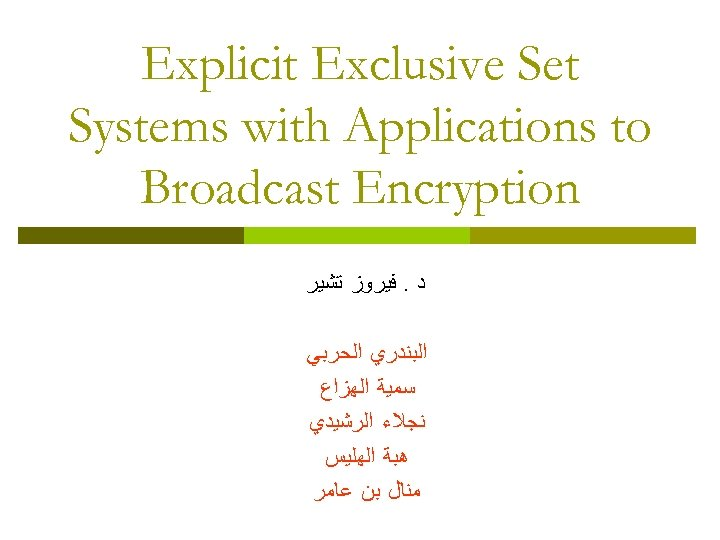Explicit Exclusive Set Systems with Applications to Broadcast Encryption ﺩ. ﻓﻴﺮﻭﺯ ﺗﺸﻴﺮ ﺍﻟﺒﻨﺪﺭﻱ ﺍﻟﺤﺮﺑﻲ ﺳﻤﻴﺔ ﺍﻟﻬﺰﺍﻉ ﻧﺠﻼﺀ ﺍﻟﺮﺷﻴﺪﻱ ﻫﺒﺔ ﺍﻟﻬﻠﻴﺲ ﻣﻨﺎﻝ ﺑﻦ ﻋﺎﻣﺮ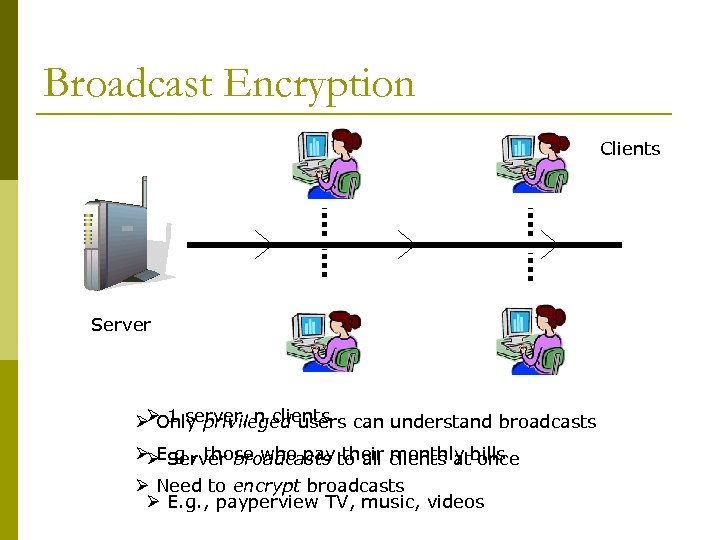Broadcast Encryption Clients Server ØØ 1 server, n clients can understand broadcasts Only privileged users ØØ Server broadcasts to all clients atbills E. g. , those who pay their monthly once Ø Need to encrypt broadcasts Ø E. g. , payperview TV, music, videos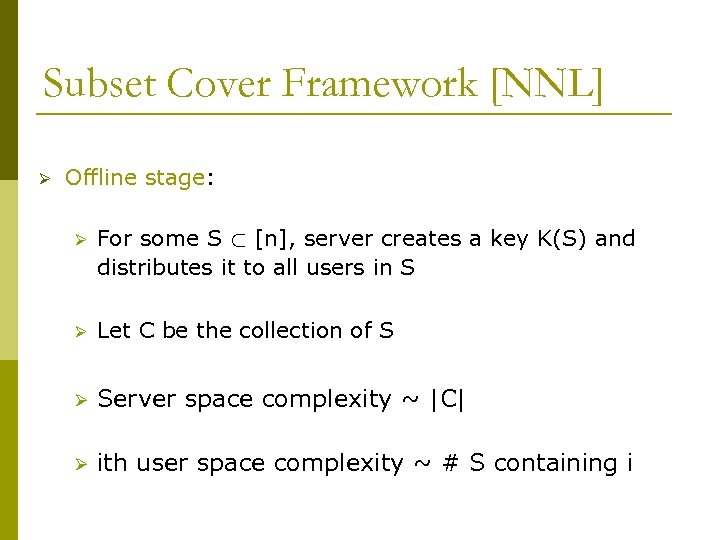Subset Cover Framework [NNL] Ø Offline stage: Ø For some S ½ [n], server creates a key K(S) and distributes it to all users in S Ø Let C be the collection of S Ø Server space complexity ~ |C| Ø ith user space complexity ~ # S containing i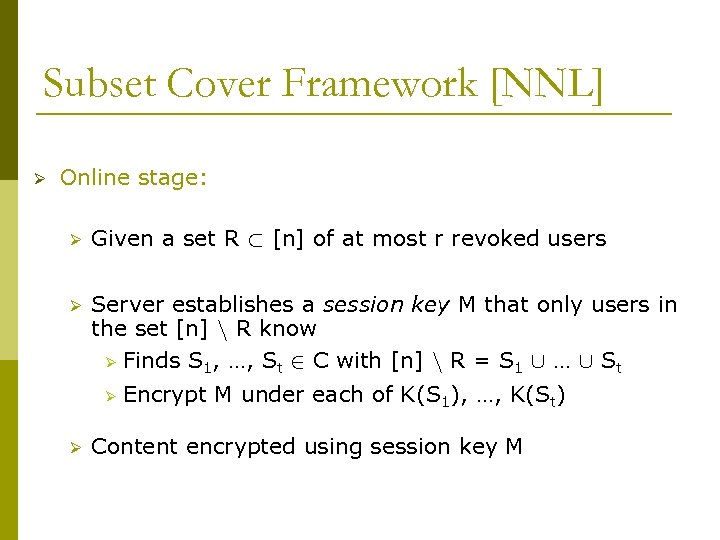Subset Cover Framework [NNL] Ø Online stage: Ø Given a set R ½ [n] of at most r revoked users Ø Server establishes a session key M that only users in the set [n] n R know Ø Finds S 1, …, St 2 C with [n] n R = S 1 [ … [ St Ø Ø Encrypt M under each of K(S 1), …, K(St) Content encrypted using session key M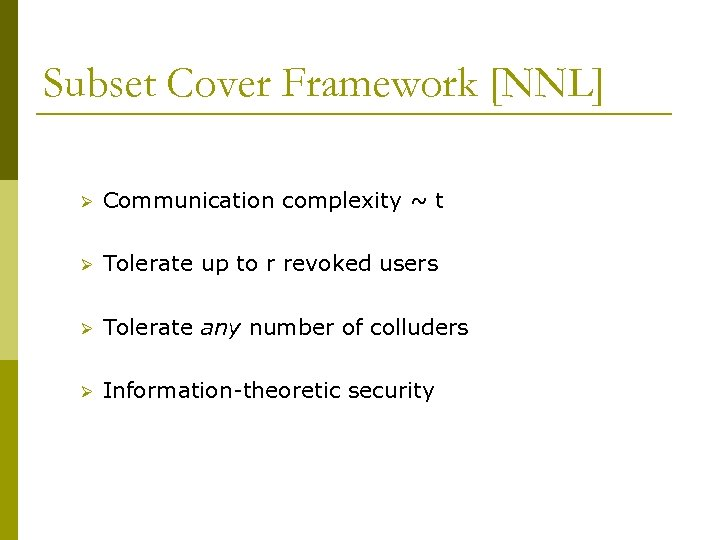Subset Cover Framework [NNL] Ø Communication complexity ~ t Ø Tolerate up to r revoked users Ø Tolerate any number of colluders Ø Information-theoretic security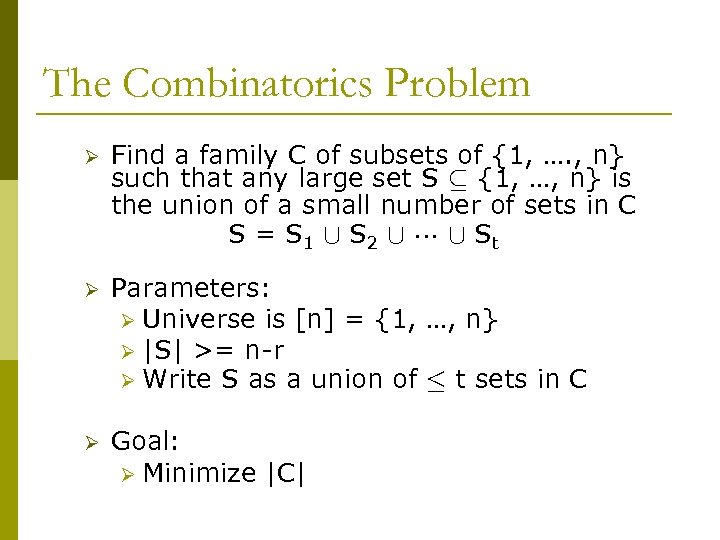The Combinatorics Problem Ø Find a family C of subsets of {1, …. , n} such that any large set S µ {1, …, n} is the union of a small number of sets in C S = S 1 [ S 2 [ [ St Ø Parameters: Ø Universe is [n] = {1, …, n} Ø |S| >= n-r Ø Write S as a union of · t sets in C Ø Goal: Ø Minimize |C|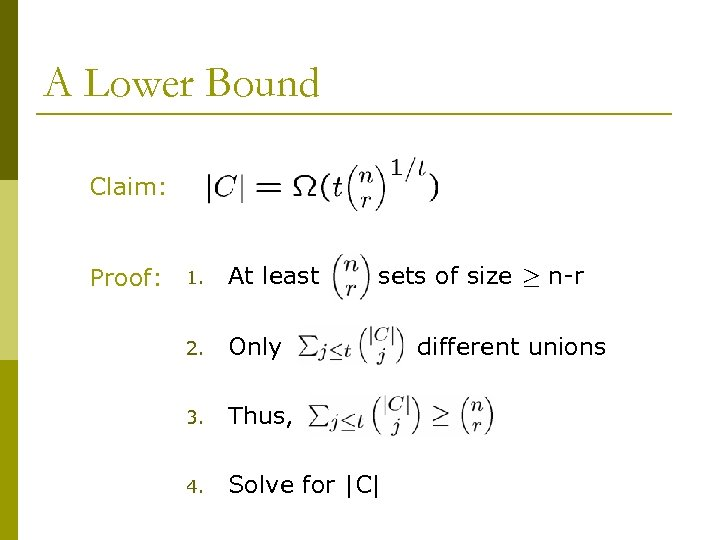A Lower Bound Claim: Proof: 1. At least sets of size ¸ n-r 2. Only 3. Thus, 4. Solve for |C| different unions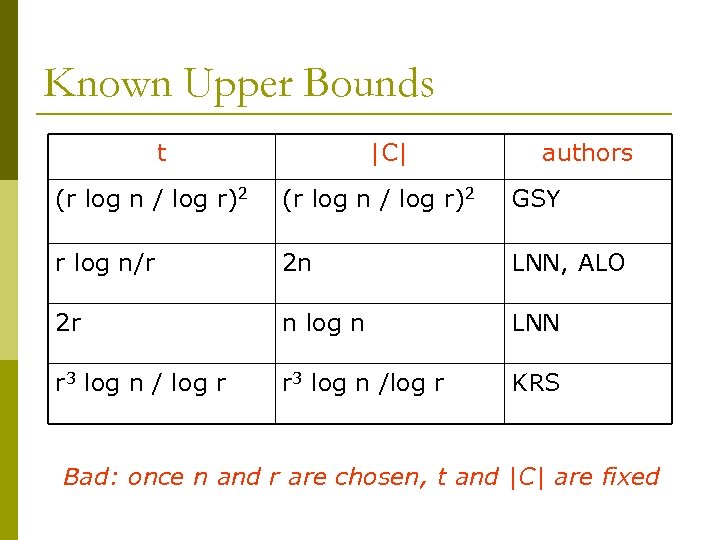Known Upper Bounds t |C| authors (r log n / log r)2 GSY r log n/r 2 n LNN, ALO 2 r n log n LNN r 3 log n / log r r 3 log n /log r KRS Bad: once n and r are chosen, t and |C| are fixed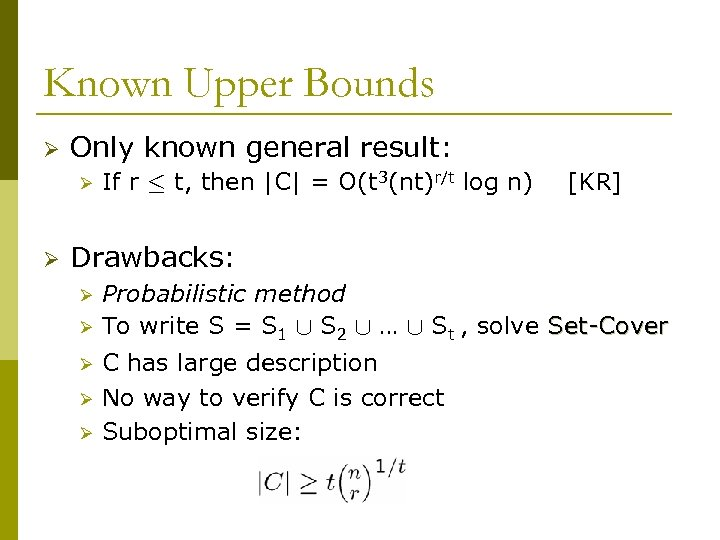Known Upper Bounds Ø Only known general result: Ø Ø If r · t, then |C| = O(t 3(nt)r/t log n) [KR] Drawbacks: Ø Ø Ø Probabilistic method To write S = S 1 [ S 2 [ … [ St , solve Set-Cover C has large description No way to verify C is correct Suboptimal size: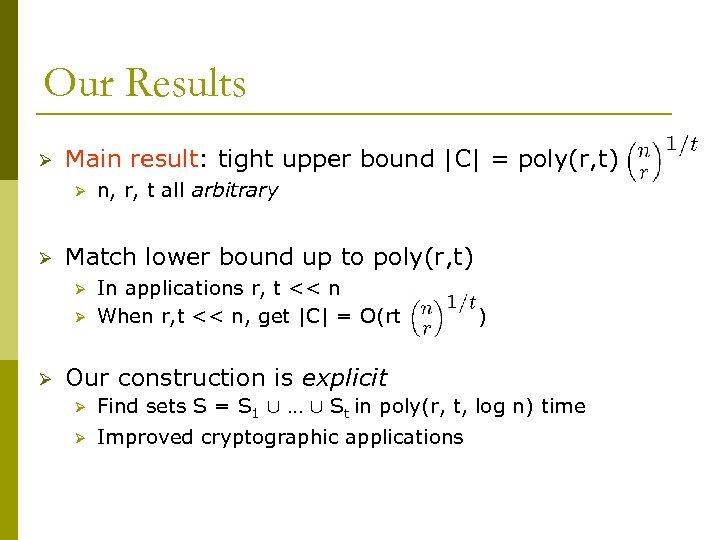Our Results Ø Main result: tight upper bound |C| = poly(r, t) Ø Ø Match lower bound up to poly(r, t) Ø Ø Ø n, r, t all arbitrary In applications r, t << n When r, t << n, get |C| = O(rt ) Our construction is explicit Ø Find sets S = S 1 [ … [ St in poly(r, t, log n) time Ø Improved cryptographic applications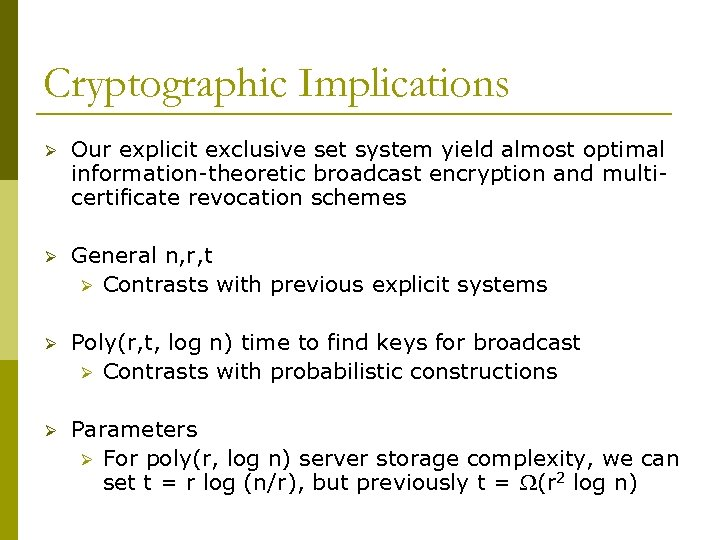Cryptographic Implications Ø Our explicit exclusive set system yield almost optimal information-theoretic broadcast encryption and multicertificate revocation schemes Ø General n, r, t Ø Contrasts with previous explicit systems Ø Poly(r, t, log n) time to find keys for broadcast Ø Contrasts with probabilistic constructions Ø Parameters Ø For poly(r, log n) server storage complexity, we can set t = r log (n/r), but previously t = (r 2 log n)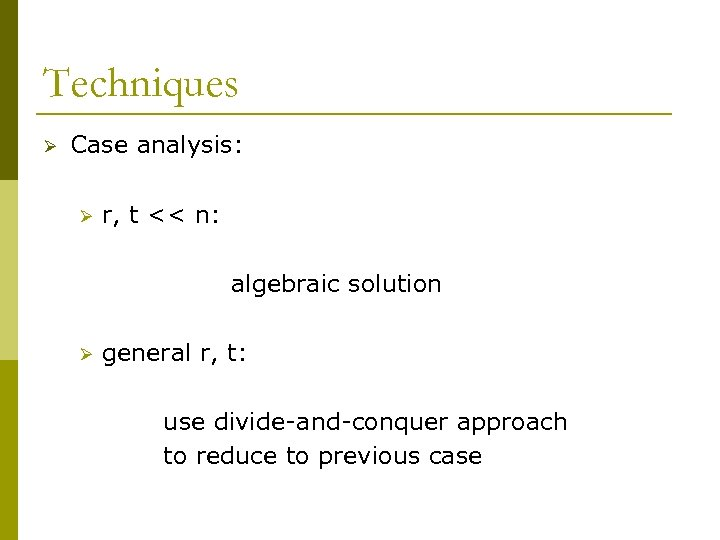Techniques Ø Case analysis: Ø r, t << n: algebraic solution Ø general r, t: use divide-and-conquer approach to reduce to previous case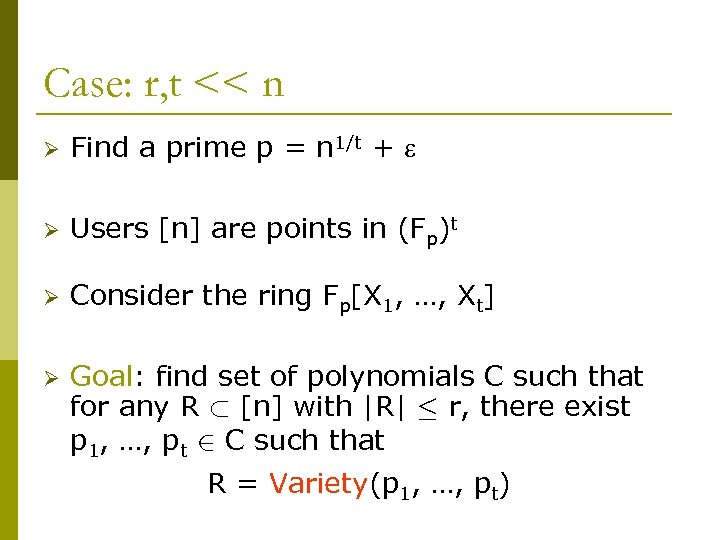Case: r, t << n Ø Find a prime p = n 1/t + Ø Users [n] are points in (Fp)t Ø Consider the ring Fp[X 1, …, Xt] Ø Goal: find set of polynomials C such that for any R ½ [n] with |R| · r, there exist p 1, …, pt 2 C such that R = Variety(p 1, …, pt)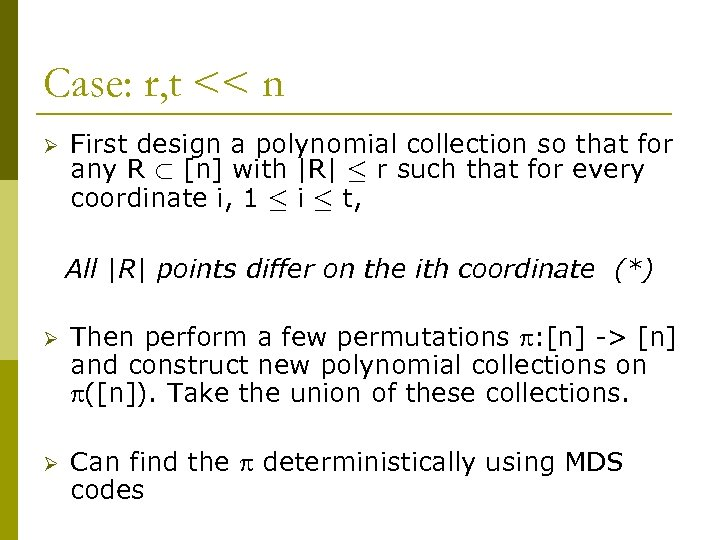Case: r, t << n Ø First design a polynomial collection so that for any R ½ [n] with |R| · r such that for every coordinate i, 1 · i · t, All |R| points differ on the ith coordinate (*) Ø Then perform a few permutations : [n] -> [n] and construct new polynomial collections on ([n]). Take the union of these collections. Ø Can find the deterministically using MDS codes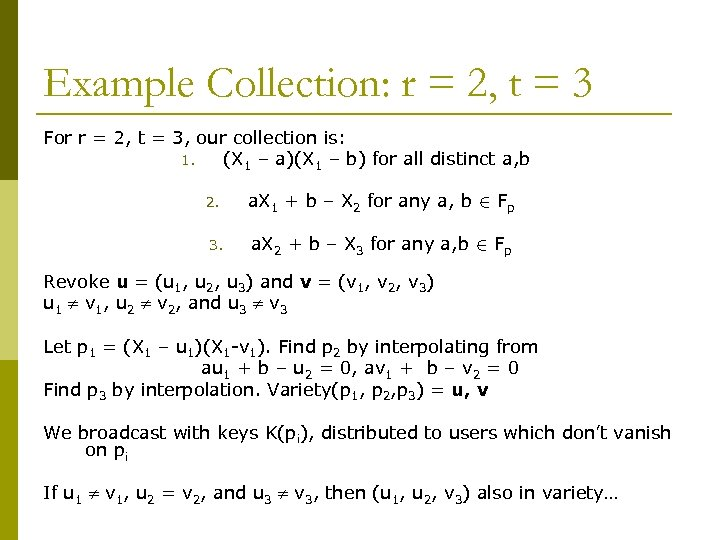Example Collection: r = 2, t = 3 For r = 2, t = 3, our collection is: 1. (X 1 – a)(X 1 – b) for all distinct a, b 2. a. X 1 + b – X 2 for any a, b 2 Fp 3. a. X 2 + b – X 3 for any a, b 2 Fp Revoke u = (u 1, u 2, u 3) and v = (v 1, v 2, v 3) u 1 v 1, u 2 v 2, and u 3 v 3 Let p 1 = (X 1 – u 1)(X 1 -v 1). Find p 2 by interpolating from au 1 + b – u 2 = 0, av 1 + b – v 2 = 0 Find p 3 by interpolation. Variety(p 1, p 2, p 3) = u, v We broadcast with keys K(pi), distributed to users which don’t vanish on pi If u 1 v 1, u 2 = v 2, and u 3 v 3, then (u 1, u 2, v 3) also in variety…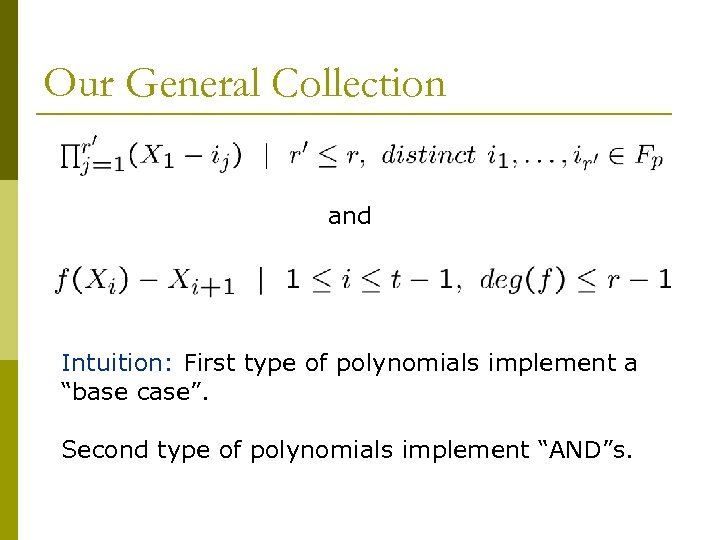Our General Collection and Intuition: First type of polynomials implement a “base case”. Second type of polynomials implement “AND”s.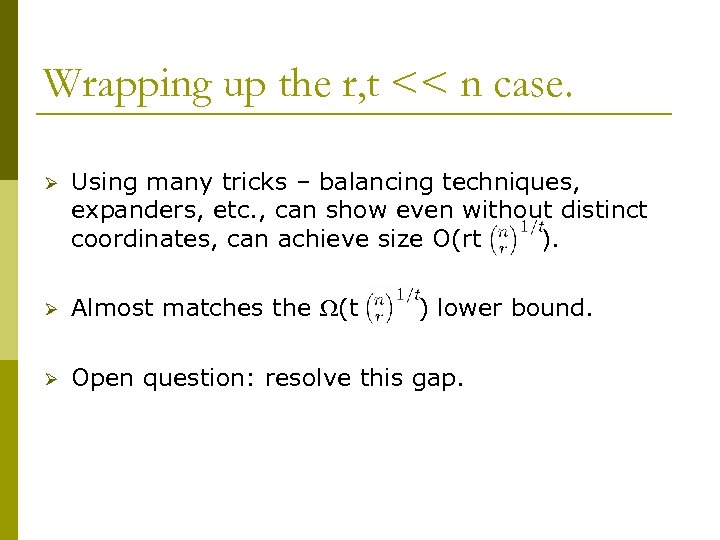Wrapping up the r, t << n case. Ø Using many tricks – balancing techniques, expanders, etc. , can show even without distinct coordinates, can achieve size O(rt ). Ø Almost matches the (t Ø Open question: resolve this gap. ) lower bound.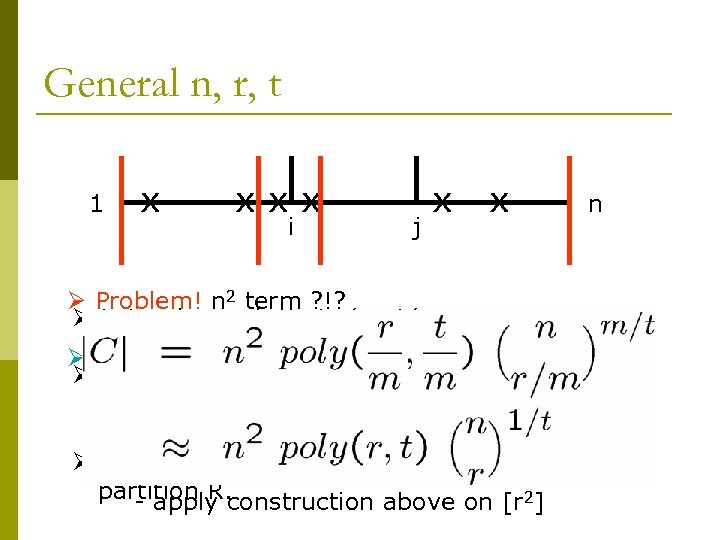General n, r, t 1 x xxx i j x x n Ø Problem! n 2 term ? !? Ø Let m be such that r/m, t/m << n Ø Fix: - hash [n] to [r 2] first Ø For every interval [i, j], form an exclusive set system enough = j-i+1, so = r/m, t’an injective - do with n’ hashes r’ there is = t/m hash for every R Ø Given a set R, find intervals which evenly partition R. - apply construction above on [r 2]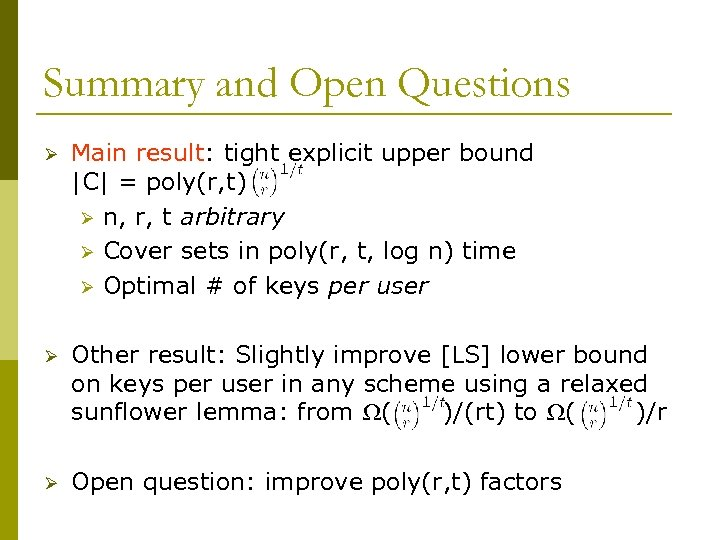Summary and Open Questions Ø Main result: tight explicit upper bound |C| = poly(r, t) Ø n, r, t arbitrary Ø Cover sets in poly(r, t, log n) time Ø Optimal # of keys per user Ø Other result: Slightly improve [LS] lower bound on keys per user in any scheme using a relaxed sunflower lemma: from ( )/(rt) to ( )/r Ø Open question: improve poly(r, t) factors